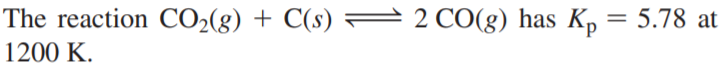×
Get Full Access to Chemistry: A Molecular Approach - 3 Edition - Chapter 14 - Problem 75e
Get Full Access to Chemistry: A Molecular Approach - 3 Edition - Chapter 14 - Problem 75e

×

# a. Calculate the total pressure at equilibrium when 4.45 gISBN: 9780321809247 1

## Solution for problem 75E Chapter 14

Chemistry: A Molecular Approach | 3rd Edition

• Textbook Solutions
• 2901 Step-by-step solutions solved by professors and subject experts
• Get 24/7 help from StudySoup virtual teaching assistantsChemistry: A Molecular Approach | 3rd Edition

4 5 1 265 Reviews
16
2
Problem 75Ea. Calculate the total pressure at equilibrium when 4.45 g of CO2 is introduced into a 10.0 L container and heated to 1200 K in the presence of 2.00 g of graphite.

b. Repeat the calculation of part a in the presence of 0.50 g of graphite.

Step-by-Step Solution:

Solution 75EStep 1Molarity is the number of moles of solute present in 1 Litre of solution.It can be calculated by the following formulae.In the above formulaeThe study of reactant and products in a chemical reaction is known as Stoichiometry. Moles areused to calculate the amount of product formed or amount of reactant required to form theproduct in the reaction, called mole method .The stoichiometric coefficients of a chemical equation are assumed as no. of moles of thesubstance as relative number of molecules are same as the relative number of moles.It is stated by Dalton's law of partial pressures that the total pressure exerted by a mixture ofgases is the sum of partial pressure of each individual gas. The gas behaves as ideal gas. It isrepresented as follows:\n

Step 2 of 5

Step 3 of 5

## Discover and learn what students are asking

Calculus: Early Transcendental Functions : Slope Fields and Eulers Method
?In Exercises 1-8, verify the solution of the differential equation. Solution D

Statistics: Informed Decisions Using Data : Tests for Independence and the Homogeneity of Proportions
?Credit Risk Traditional underwriting to determine the risks associated with lending include credit scores, income, and employment history. The online

Statistics: Informed Decisions Using Data : Comparing Three or More Means (One-Way Analysis of Variance)
?True or False: The F-test statistic is F0 = MST/MSE.

Unlock Textbook Solution